Function Repository Resource:

NotchedPolygon

Represent a polygon with notched corners

Contributed by: Jan Mangaldan
 ResourceFunction["NotchedPolygon"][{p1,…,pn},r] represents a filled notched polygon with points pi and notch radius r. ResourceFunction["NotchedPolygon"][{p1,…,pn},{r1,…,rn}] represents a filled notched polygon with points pi and corresponding notch radii ri.

Details

ResourceFunction["NotchedPolygon"] returns a Polygon object, and can be used as a graphics primitive.
ResourceFunction["NotchedPolygon"][poly,rspec], where poly is a Polygon, Rectangle or Triangle object, gives a notched version of poly.
The notch radii ri must be non-negative numbers.
ResourceFunction["NotchedPolygon"] works with 2D and 3D polygons.

Examples

Basic Examples (2)

A triangle with notched corners:

 In:=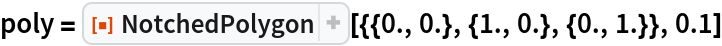Out=In:=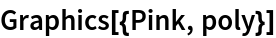Out=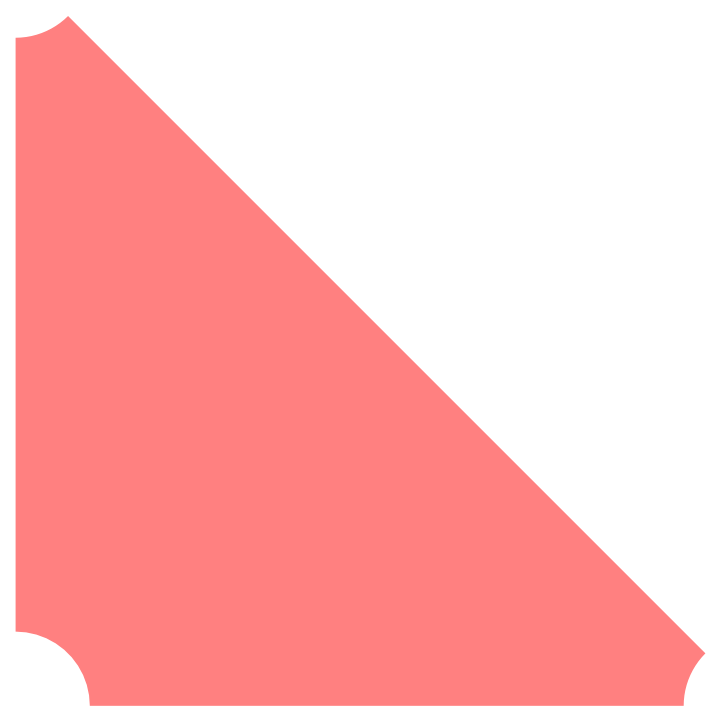A rectangle with different notch radii for each corner:

 In:=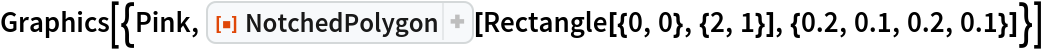Out=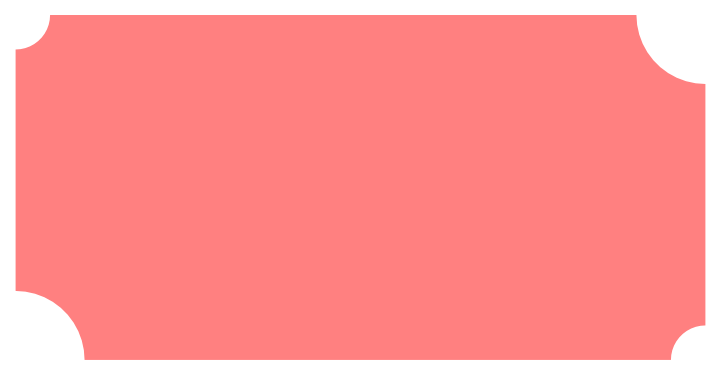Scope (4)

Coordinates for a star-shaped polygon:

 In:=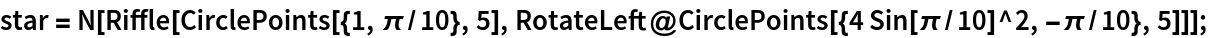Show the original polygon and the notched version:

 In:=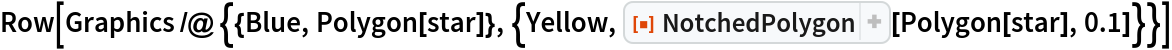Out=Use different notch radii for each vertex:

 In:=Out=A notched 3D polygon:

 In:=Out=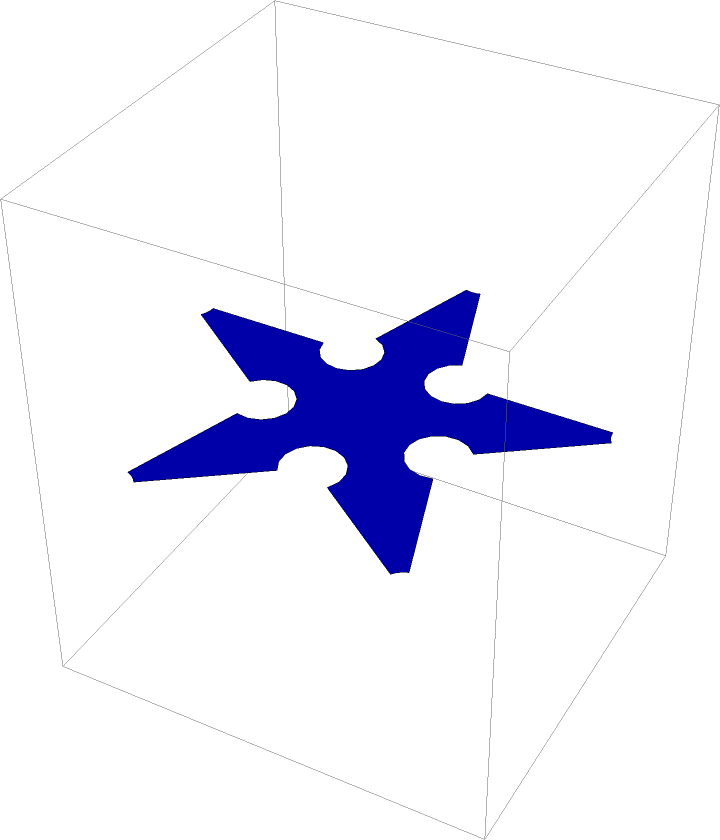Applications (2)

Plot a function over a notched polygon domain:

 In:=Out=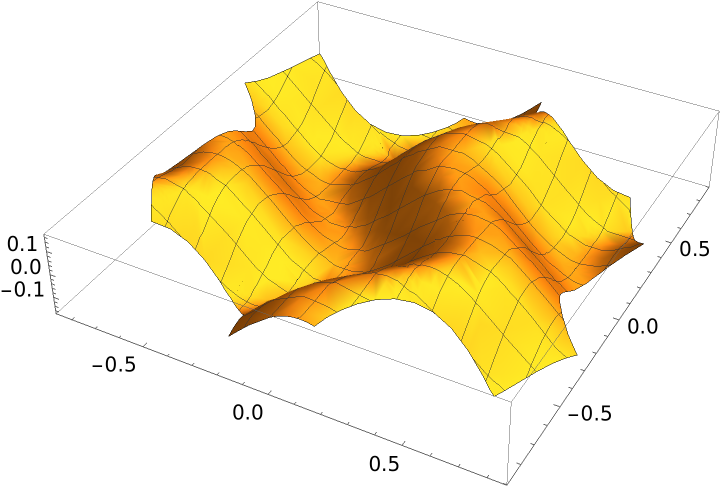A polyhedron with notched faces:

 In:=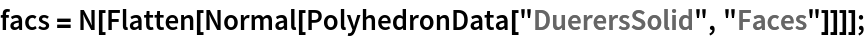In:=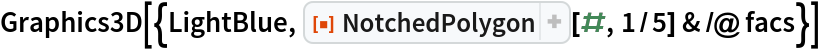Out=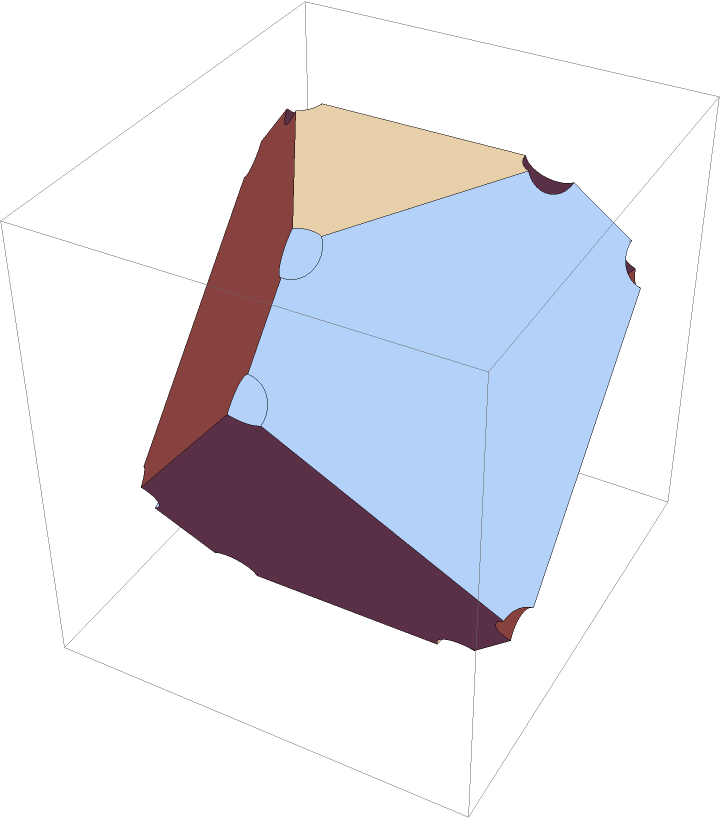Properties and Relations (1)

NotchedPolygon returns a Polygon object:

 In:=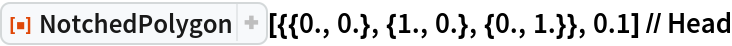Out=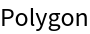Possible Issues (2)

If the notch radius is too large, NotchedPolygon may give unexpected results:

 In:=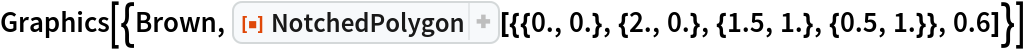Out=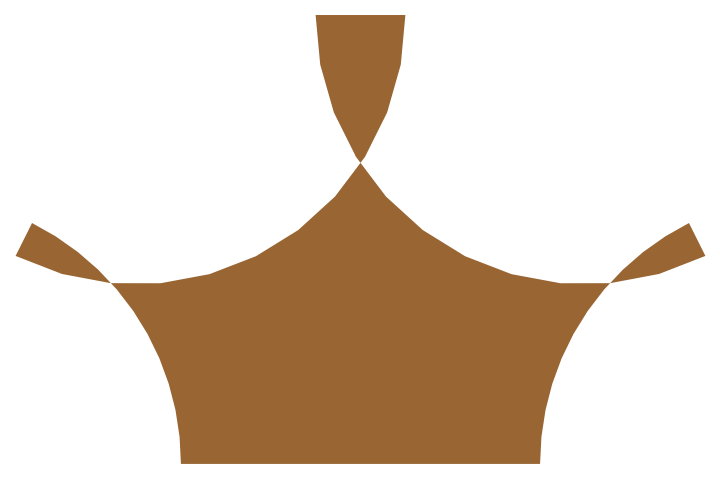In:=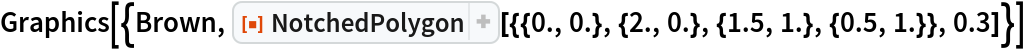Out=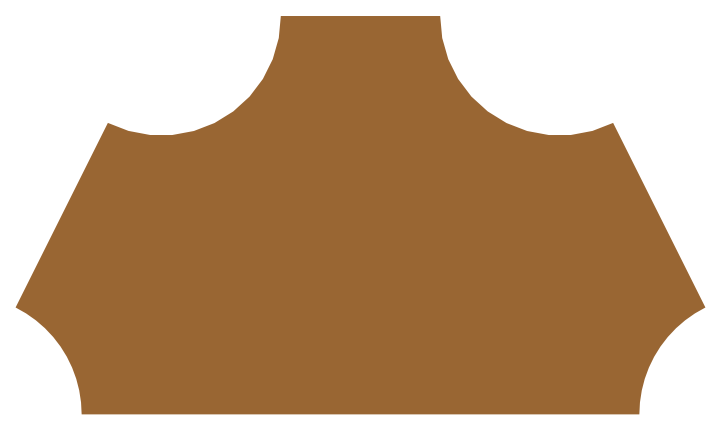Neat Examples (1)

Use NotchedPolygon with the resource function OutlinePolygons on a truncated icosahedron:

 In:=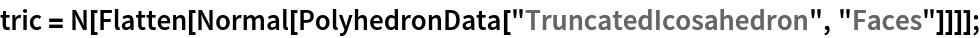In:=Out=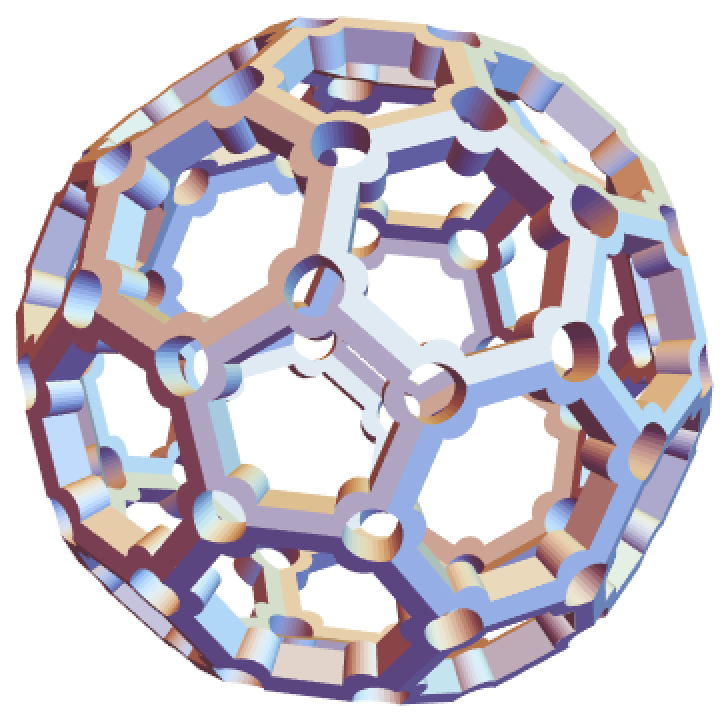Author Notes

Polygons with holes are currently not supported.